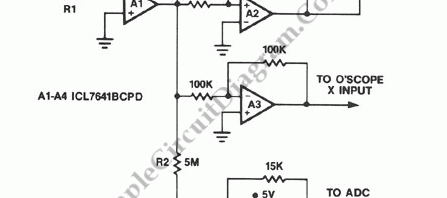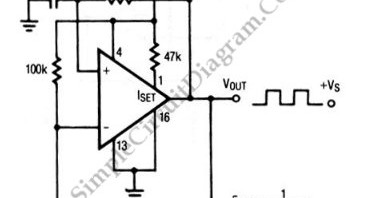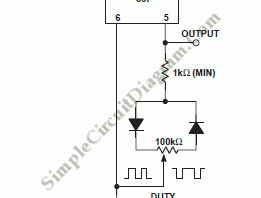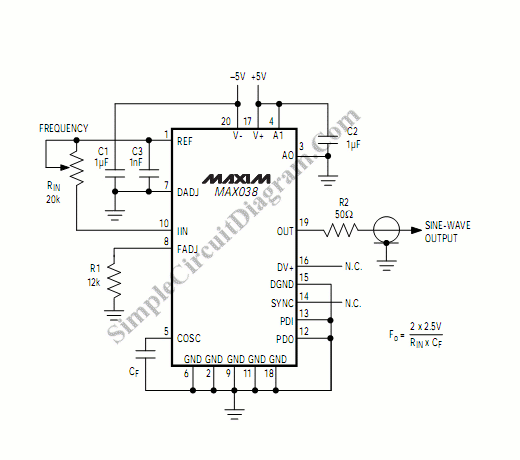## Triangle Waveform Signal GeneratorA symmetrical, 10 mV peak to peak triangle waveform can be generated by the circuit depicted in the following schematic diagram. This circuit is featured with alternative DC biased output which can be used to feed an ADC.  The DC level input offsets the triangle waveform over the input range of the ADC.

## Square Wave Oscillator using Comparator/Op-AmpThe circuit shown in this schematic diagram is a square-wave oscillator circuit.  The main component of this oscillator circuit is LP165/365 comparator. As timing component, it uses capacitor and resistor to determine the frequency operation, generating square waves signal. Here is the schematic diagram of the circuit: We can change the values of R and C to produce different frequencies. […]

## Adjustable Duty Cycle 567 IC Pulse GeneratorPulse generator circuit shown in the schematic diagram below produces square wave pulse with adjustable duty cycle/active factor. The benefits of using this circuit are low components count and high stability of its output frequency. The frequency of this pulse generator is fixed, the frequency f depends on the value of R and C1, following the formula of  f=1/(1.1*R1*C1), where […]